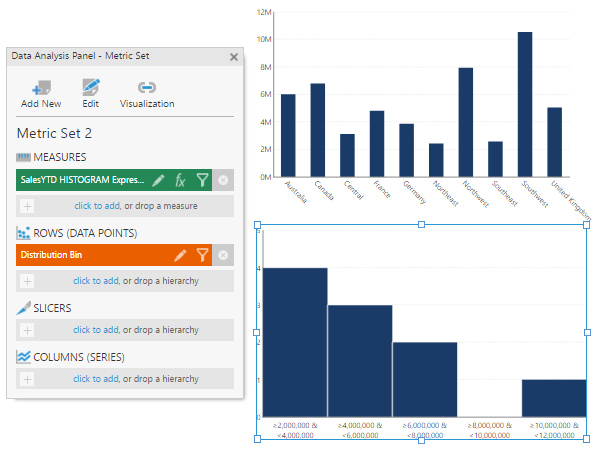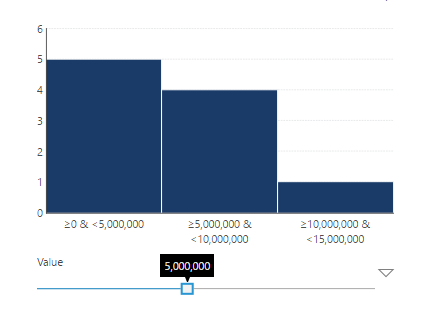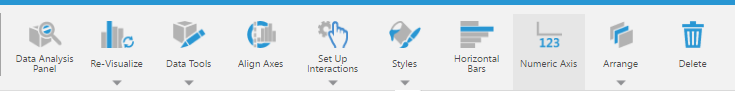Calculate histogram

Contents[Hide]

1. Overview

A histogram is a graphical representation of the distribution of numerical data, grouping each value into a "bin" of values and displaying the bin counts across the range of values. Dundas BI can automatically create a formula visualization that calculates and displays the frequencies of values in your dataset.

The Calculate Histogram data visualization automatically generates a formula visualization based on the Histogram formula. To create the formula visualization manually, refer to the article Histogram article.

When to use a histogram?

Use a histogram when you have a single set of numeric values and are interested in which numeric values occur the most or least, and patterns in how the values occur across the total range.

2. Data preparation

The following example relies on the Adventure Works database. From the [Sales].[SalesTerritory] table, create a data visualization with SalesYTD as a measure and TerritoryID as the hierarchy (on the Rows field of the Data Analysis Panel). Drag Name over TerritoryID to display the territory names.

In this example, you have data showing sales per location, and you want to show in a data visualization the distribution of the frequency of different amount of sales.

3. Creating the Histogram

With your data visualization selected, select Re-Visualize from the toolbar or the context menu, and then select Calculate Histogram.

A new bar chart is created, with the histogram formula as the measure (the height of the bars corresponds to the frequency of the amount of data) and Distribution Bin as the hierarchy and the bottom axis.The Histogram is created as a new bar chart

Note
Another method is to start by creating a Calculate Histogram visualization. After dropping the measure and hierarchy onto the Data Analysis Panel, click Create Histogram from the toolbar.

4. Adding a filter to dynamically change the distribution bin

By default, the bin size parameter of the Histogram formula is assigned a parameter placeholder called \$BinSizeParameter\$. This makes it possible to assign this parameter placeholder to a single numeric parameter so that users can dynamically set the bin size. The sample below demonstrates how to add a single numeric filter (i.e. Slider) and connect to the \$BinSizeParameter\$ placeholder parameter. A Single Number filter can also be used.

1. Add a Slider filter.

2. Assign to the "(SingleNumber)" parameter under the "Sales Histogram Expression" measure.

Note
If this is the first filter you are adding to the dashboard, the Slider will be treated as a range and SingleNumber will not be available. It will appear as an option once you uncheck all of the auto-selected parameters.

3. Set up the Slider properties.

View the dashboard and use the slider control.Use the slider control in View mode

5. Configuring the parameters

If you prefer to explicitly set the parameter values, you can modify the formula to set the histogram's distribution bin and format. For details about the parameters in this formula, refer to the Histogram Article.

In the Data Analysis Panel for the Histogram, edit the measure (click the fx icon next to it).

Replace the parameter placeholder with your preferred bin size, and indicate the format.

Optionally, you can use the Histogram Range function to set the start and end range of the distribution bins:

6. Changing the axis

By default, the bottom axis of a Histogram visualization is set to a category scale. The axis labels for a category scale are positioned under the middle of each bin and indicate the range of values in that bin (for example, 2,000,000s or >=0 & < 5,000,000).

You can quickly change this representation to a numeric scale if you want the axis labels to be located at the edges of the bins instead. Simply select Numeric Axis from the toolbar.Select Numeric Axis from the toolbar

Dundas Data Visualization, Inc.
500-250 Ferrand Drive